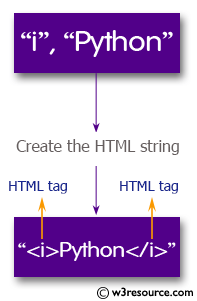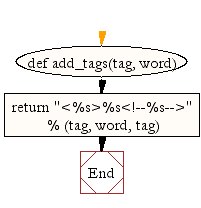﻿ Python: Create the HTML string with tags around the word(s) - w3resource# Python: Create the HTML string with tags around the word(s)

## Python String: Exercise-15 with Solution

Write a Python function to create the HTML string with tags around the word(s).
Sample function and result :
add_tags('b', 'Python Tutorial') -> '<b>Python Tutorial </b>'Sample Solution:-

Python Code:

``````def add_tags(tag, word):
return "<%s>%s</%s>" % (tag, word, tag)
```
```

Sample Output:

```Python
Python Tutorial
```

Flowchart:Python Code Editor:

Have another way to solve this solution? Contribute your code (and comments) through Disqus.

What is the difficulty level of this exercise?

Test your Programming skills with w3resource's quiz.

﻿

## Python: Tips of the Day

Performs right-to-left function composition:

Example:

```from functools import reduce

def tips_compose(*fns):
return reduce(lambda f, g: lambda *args: f(g(*args)), fns)
add6 = lambda x: x + 6
multiply = lambda x, y: x * y
```36